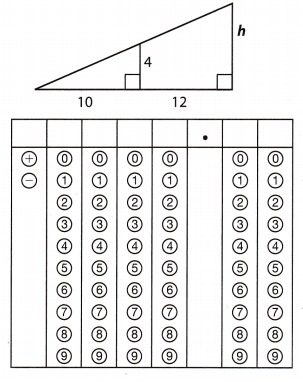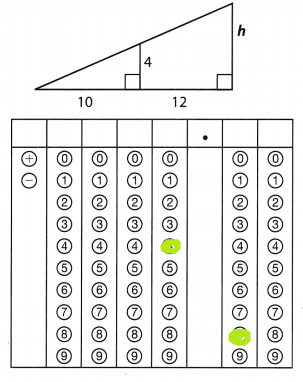Refer to our Texas Go Math Grade 8 Answer Key Pdf to score good marks in the exams. Test yourself by practicing the problems from Texas Go Math Grade 8 Module 7 Quiz Answer Key.

7.1 Parallel Lines Cut b a Transversal

In the figure, line P|| line q. Find the measure of each angle if m∠8 = 115°.

Question 1.
m∠7 = ______________
According to the Exterior Angle Theorem, we have:
m∠7 + m∠8 = 180°
m∠7 + 115° = 180°
m∠7 + 115° – 115° = 180° – 115°
m∠7 = 65°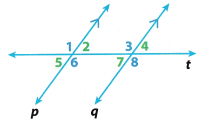Question 2.
m∠6 = ______________In the given figure, line P is parallel to line q. So, the angles given in line P is equal to the angles in line q.(corresponding angles)
so, m∠8 is parallel to m∠6
or m∠8 = m∠6 = 115°

Question 3.
m∠1 = ______________this is the given figure of the question

taking a section of a figure, we haveIn the previous question, we got m∠6 = 115°
In the figure, ∠1 and ∠6 are alternate exterior angles
so, m∠1 = m∠6 = 115°

7.2 Angle Theorems for Triangles

Find the measure of each angle.

Question 4.
m∠A = ______________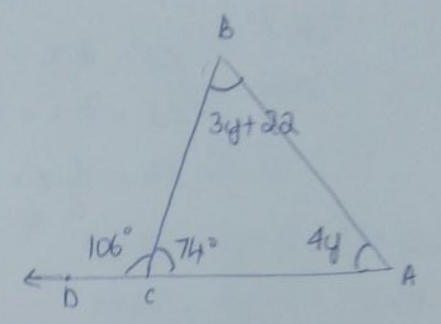From the triangle sum theorem
sum of the angles of triangle is 180°
m∠A + m∠B + m∠C = 180°
4y + (3y + 22) + 74° = 180°
7y = 180 – 96 = 84
y = 12°

m∠A = 4y = 4 × 12 = 48°
and m∠B = 3y + 22 = 3 × 12 + 22
= 58°Question 5.
m∠B = ________
Using the Exterior Angle Theorem we have:
m∠A + m∠B = m∠BCD
We substitute the given angle measures and we solve the equation for y.
(4y)° + (3y + 22)° = 106°
4y° + 3y° + 22° = 106°
7y° + 22° = 106°
7y° + 22° – 22° = 106° – 22°
7y° = 84°
$$\frac{7 y^{\circ}}{7^{\circ}}$$ = $$\frac{84^{\circ}}{7^{\circ}}$$
y = 12
We use the value of y to find m∠B.
m∠B = (3y + 22)° = (3 . 12 + 22)° = 58°
m∠B = 58°

Question 6.
m∠BCA = ________In the given triangle,
m∠A = 4y m∠B = 3y + 22°
and m∠BCD = 106°
using exterior angle theorem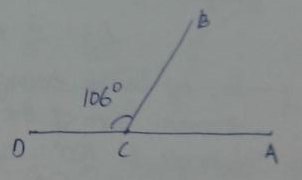m∠BCD + m∠BCA = 180°
m∠BCA = 180° – 106°
= 74°
So, m∠BCA = 74°

∠BCA = 74 degrees

7.3 Angle-Angle Similarity

Triangle FEG is similar to triangle IHJ. Find the missing values.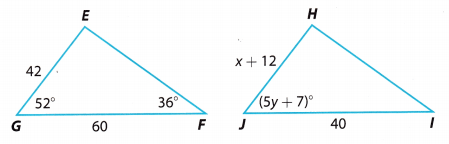Question 7
x = ________
In similar triangles, corresponding side lengths are proportional
$$\frac{H J}{E G}$$ = $$\frac{I J}{F G}$$
$$\frac{x+12}{42}$$ = $$\frac{40}{60}$$
$$\frac{x+12}{42}$$ = $$\frac{4}{6}$$
6 . 42 . $$\frac{x+12}{42}$$ = $$\frac{4}{6}$$ . 6 . 42
6(x + 12) = 168
6x + 72 = 168
6x + 72 – 72 = 168 – 72
6x = 96
$$\frac{6 x}{6}$$ = $$\frac{96}{6}$$
x = 16

Question 8.
y = ________
In similar triangles, corresponding angles are congruent
m∠HJI = m∠EGF
(5y + 7)° = 52°
5y + 7° = 52°
5y° + 7° – 7° = 52° – 7°
5y° = 45
$$\frac{5 y^{\circ}}{5^{\circ}}$$ = $$\frac{45^{\circ}}{5^{\circ}}$$
y = 9

Question 9.
m∠H = ___
From the Triangle Sum Theorem we have:
m∠E + m∠F + m∠G = 180°
We substitute the given angle measures and we solve for m∠E.
m∠E + 36° + 52° = 180°
m∠E + 88° = 180°
m∠E + 88° – 88° = 180° – 88°
m∠E = 92°
In similar triangles, corresponding angles are congruent. Therefore,
m∠H = m∠E
m∠H = 92°

Essential Question

Question 10.
How can you use similar triangles to solve real-world problems?
We know that if two triangles are similar, then their corresponding angles are congruent and the lengths of their
corresponding sides are proportional. We can use this to determine values that we cannot measure directly. For example, we can calculate the length of the tree if we measure its shadow and our shadow in a sunny day.

Texas Go Math Grade 8 Module 7 Mixed Review Texas Test Prep Answer Key

Selected Response

Use the figure for Exercises 1 and 2.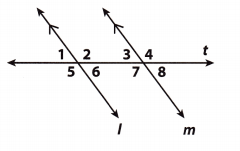Question 1.
Which angle pair is a pair of alternate exterior angles?
(A) ∠5 and ∠6
(B) ∠6 and ∠7
(C) ∠5 and ∠4
(D) ∠5 and ∠2
(C) ∠5 and ∠4

Explanation:
∠5 and ∠4 are alternate exterior angles

Question 2.
Which of the following angles is not congruent to ∠3?
(A) ∠1
(B) ∠2
(C) ∠6
(D) ∠8
(B) ∠2

Explanation:
∠2 and ∠3 are same-side interior angles. They are not congruent; instead their sum is equal to 180°

Question 3.
The measures of the three angles of a triangle are given by 2x + 1, 3x – 3, and 9x. What is the measure of the smallest angle?
(A) 13°
(B) 27°
(C) 36°
(D) 117°
(B) 27°

Explanation:
From the Triangle Sum Theorem we have:
m∠1 + m∠2 + m∠3 = 180°
We substitute the given angle measures and we solve for X.
(2x + 1)° + (3x – 3)° + (9x)° = 180°
2x° + 1 + 3x° – 3° + 9x° = 180°
14x° – 2° = 180°
14x° – 2° + 2° = 180° + 2°
14x° = 178°
$$\frac{14 x^{\circ}}{14^{\circ}}$$ = $$\frac{182^{\circ}}{14^{\circ}}$$
x = 13
We use the value of x to find m∠1, m∠2 and m∠3.
m∠1 = (2x + 1)° = (2 . 13 + 1)° = 27°
m∠1 = 27°
m∠2 = (3x – 3)° = (3 . 13 – 3)° = 36°
m∠2 = 36°
m∠3 = (9x)° = (9 . 13)° = 117°
m∠3 = 117°
The smallest angle is 27°

Question 4.
Which is a possible measure of ∠DCA in the triangle below?
(A) 36°
(B) 38°
(C) 40°
(D) 70°
(D) 70°

Explanation:
Using the Exterior Angle Theorem we have:
m∠A + m∠B = m∠DCA
m∠A + 40° = m∠DCA
As we can see, m∠DCA will be greater than 40°. The only suitable option is D, 70°.

Question 5.
Kaylee wrote in her dinosaur report that the Jurassic period was 1.75 × 108 years ago. According to Kaylee’s report, how many years ago was the Jurassic period?
(A) 1,750,000
(B) 17,500,000
(C) 175,000,000
(D) 17,500,000,000
(C) 175,000,000

Explanation:
The Jurassic period was 1.75 × 108 years ago. In standard form
108 = 100, ooo, 000 (Ten with eight zeros) (1)
1.75 × 108 = 1.75 × 100, 000,000 (Substitute) (2)
= 175,000,000 (3)

Correct answer is C 175, 000, 000

Question 6.
Given that y varies directly with x, what is the equation of direct variation if y is 16 when x is 20?
(A) y = 1$$\frac{1}{5}$$x
(B) y = $$\frac{5}{4}$$x
(C) y = $$\frac{4}{5}$$x
(D) y = 0.6x
(C) y = $$\frac{4}{5}$$x

Explanation:
Given that y is proportional to x, and if y is 16, and x is 20 we can write proportion
$$\frac{y}{x}$$ = $$\frac{16}{20}$$ (1)
$$\frac{y}{x}$$ = $$\frac{4}{5}$$x (simplify the fraction) (2)
x × $$\frac{4}{5}$$ = $$\frac{4}{5}$$ × x (Use properties of equality to get y by itself.) (3)
y = $$\frac{4}{5}$$x (4)

Gridded Response

Question 7.
What is the value of h in the triangle below?